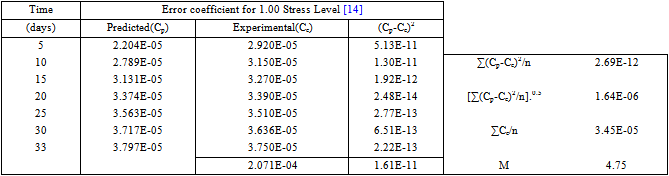﻿ Simplified Parametric (Phenomenological) Model of Creep of Concrete in Direct Tension

Simplified Parametric (Phenomenological) Model of Creep of Concrete in Direct Tension

Ephraim M. E., Rowland-Lato E. O.OPEN ACCESSPEER-REVIEWED

Simplified Parametric (Phenomenological) Model of Creep of Concrete in Direct Tension

Ephraim M. E.1, Rowland-Lato E. O.2,1Department of Civil Engineering, Rivers State University of Science and Technology, Port-Harcourt, Nigeria

2Department of Civil Engineering, University of Port Harcourt, Port Harcourt, Nigeria

Abstract

An analytical model based on the experimental trend of the creep deformation of concrete in direct tension is developed. The model is calibrated for normal and high strength concretes and the results show close agreement with experimental data. The inclussion of the stress level is a unique feature of the model and presents a prospect for predicting concrete deformation for any regime of loading. The model confirm that the creep strain of concrete in tension are non-linear right frm the begining of loading therby ruling out the applicability of the principle of superposition The model presents a means for incorporating the nonlinearlity of creep into other predictive models of concrete.

At a glance: Figures

1
Prev Next

• E., Ephraim M., and Rowland-Lato E. O.. "Simplified Parametric (Phenomenological) Model of Creep of Concrete in Direct Tension." American Journal of Civil Engineering and Architecture 3.2 (2015): 52-58.
• E., E. M. , & O., R. E. (2015). Simplified Parametric (Phenomenological) Model of Creep of Concrete in Direct Tension. American Journal of Civil Engineering and Architecture, 3(2), 52-58.
• E., Ephraim M., and Rowland-Lato E. O.. "Simplified Parametric (Phenomenological) Model of Creep of Concrete in Direct Tension." American Journal of Civil Engineering and Architecture 3, no. 2 (2015): 52-58.

 Import into BibTeX Import into EndNote Import into RefMan Import into RefWorks

1. Introduction

The complex behaviour of concrete, arising from the composite heterogeneous nature of its internal structure is difficult to model. This calls for the development of appropriate constitutive models for its deformation under load. Consequently, the existing creep models have been developed with increasing levels of complexity in a bid to reflect the complete features of the material. Thus, several theories and hypotheses based on various perceptions about the concrete internal structure have been propounded for the explanation of the mechanism of the creep phenomenon in concrete.

Generally, the study of creep under compression has received significantly greater attention of researchers than of its behaviour under tensile loading. The early functional formulations were derived from two fundamental theories: the hereditary theories and he theory of ageing. The basic mathematical espression from these theories espressed as afunction of stress level,age at loading and duration of loading have the form of integral equations [2, 3, 4, 5, 6, 7, 8, 18].

In addition, there are few approximations of laboratory experimental data in use for quick assessment of creep deformations in design [1, 2, 9, 10]. Recently, the contribution of micro cracking in the overall mechanism of resistance and deformation of concrete has been given greater recognition.These studies have resulted in the formulation of various three dimensional models of creep deformation of concrete in tension [2, 7, 8, 13, 20]. The interesting features of the recent models are described in the following section

(a) Three-dimessional Rheological Model

The Ephraim-Yaschuk Model  is in the form of a regular three dimensional rheological lattice, representing the concrete microvolume. Micro-fracture is represented by the continuous depletion of the bond cross sections, resulting from outage of broken weaker bond fibres, as loading is progressed with time. The resulting equations of longitudinal creep deformations are in the form of a system of ordinary homogeneous differential equations. The model allows the prediction of transverse creep strains and Poisson’s effect as a consequence of its spatial configuration.

(b) The Stochastic Three-dimensional Finite Element Models.

These include the numerical concrete models of fracture mechanics with incorporation of microprestress and solidification processes attributable to an ageing medium such as concrete [2, 3, 4, 5, 6, 7, 20]. A recent extension of these models has resulted in the confinement shear lattice model . The creep equations from these models are complex and ammenable only to computer applications.

Thus, in spite of the great contributions of the above theories and models, their practical application in structural design is difficult, for example, in the calculation of deformations and cracks at the serviceability limit state. The authors therefore recognise the need for simpler, perhaps more reliable creep models At the same time, the paucity of models of concrete creep behaviour under tensile stress is recognised,and this paper is dedicated to filling that gap.

2. Creep of Concrete under Direct Tension

As observed earlier, technical literature reveals a dire paucity of experimental work on concrete in uniaxial tension. This is associated with known technological difficulties, including gripping problems, presence of imperfections and difficulty of applying a purely concentric pull among others. It is for these reasons that several researchers have recognised the modeling option as a more perspective approach [2-9,12,13,14,15]. None-the-less, the few authoritative researches on tensile concrete [1, 13, 21] have produced invaluable data on the magnitude and variational trend of creep strain in concrete under axial tensile loading.

Among these are the extensive experimental studies of Karapetyan reviewed by Ephraim in is work on creep of normal concrete under varying stress levels  and duration of loading and on recent experimental studies on creep of high strength concrete under axial tension is reported in the publication of Hans-Wolf Reinhardt, Tassilo Rinder . Further, the various factors influencing the stress strian relation of concrete in uniaxial tension are investigated by Komlos . The accumulated experimental evidence it may be concluded that creep strains in concrete depend principally on the age at loading, the stress level applied and the duration of loading.

The features of a typical creep curve can be devided into three distinct segments as shown in the curve 1 of Figure 1.

Segment I: an initial period of rapid increase in creep deformation immediately after load application when the creep rate tends to infinity;

Segment III: a final period of renewed rapid increase just before failure of the material.

Figure 1. Plot of total Creep strain versus time(Curve 1) and stress level(Curve 2)

Although various non-linear effects are observed to be associated with the creep of concrete, concrete is generally considered as an aging linear viscoelastic material in the range of service stresses [2, 3, 4, 5]. Consequently, the common basis for most of the proposed creep models in the literature is the assumption of creep linearity, i.e. creep strains under constant stresses are assumed to be linearly related to the stress level. Meanwhile, Ostergaard, Altoubat and Stang  in their recent experimental study have confirmed that the relationship between early age tensile creep and stress level is nonlinear. This further cofirms the results of Karapetyan and Kotikyan as reviewed by Ephraim .

3. The Proposed Model of Tensile Creep

The proposed model is developed in accordance with the requirements of RILEM  regarding the identification of physical varibles, theoritical formulation and validation for prediction models fro creep and shrinkage of concrete. The most important of these includes:

(1) After optimizing its coefficients, the model should be capable of providing close fits of the individual test data covering a broad range of times, ages, humidifies, thicknesses, etc.;

(2) The model should have a rational, physically justified theoretical basis, and

(3) Should allow good and easy extrapolation of the short-time tests into long times, high ages, large thicknesses etc.

On the basis of the general trends of creep curves of Figure. 1, the following assumptions are adopted in the formulation of the proposed model.

(i) The functional dependence between creep deformaton (εc) and the duration of loading (t-τ) may be approximated by a logarithmic function of the form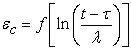(1)

Where, t - age of concrete,

λ- Time scaling factor =1 day

(ii) In line with the observation of [13, 19], the functional relationship between creep strains (εc) and the stress level applied η may be approximated by an exponential function as follows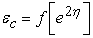(2)

Where, η = stress level defined as the ratio of applied stress to 28-day tensile strength of concrete.

(iii) The concrete material is considered unageing for which the effects of technological and environmental factors as well as micro-prestress may safely be neglected

Thus, the significant independent variables for modelling are; the tensile strength of concrete, the applied stress ltvel (η), the duration of load application (t - τ) and the initial modulus of elasticity of concrete.

(iv) The basic creep is assumed proportional to the product of the two functions.The creep strain may be represented in the form.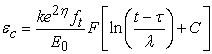(3)

where, k is model parameter depending on the strength of concrete and

determined by calibration;

C - calibration constant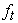- the 28-day tensile strength of concrete

Eo - the age-independent elastic modulus of concrete.

Equation 3 shows the proposed model for thereep strain of concrete,As mentioned earlier, experimental data on direct tensilecreep are scarse.However,the author have relied on the experimental resulsts published by Karapetyan and Kotikyan as reviewed by Ephraim  for creep of normal concrete and Hans-Wolf Reinhardt, Tassilo Rinder  for high strength concretefor calibration and validation of the proposed model inline with RILEM guidelines .

4. Model Calibration

The principle of least squares enables the fitting of a polynomial of any degree to achieve a close functional relationship between variables. The constants k and C were determined using the least square method for curve-fitting.

The general polynomial equation is given as:(4)

A typical creep curve for a given concrete and stress level represents asymptotic logarithmic functions (Figure 1) and can be linearized in the form of first degree polynomial with two constants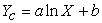(5)

Rearranging equation 3, we have: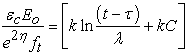(6)

If D = kC then, equation 6 becomes: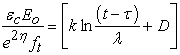(7)

Adopting the first degree polynomial, equation 4 can be reduced to: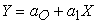(8)

The constant are determined by solving the resultant simultaneous equations presented in the following matrix form: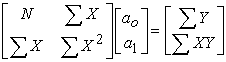(9)

Where,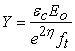,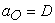, x =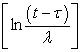and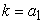.

5. Model Validation

The accuracy of the model was evaluated in terms of an error coefficient M suggested by Neville and Meyers et al :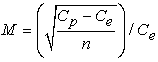(10)

Where

Cp = predicted creep from measured creep after t days under load

Ce = actual creep after t days under load;

n = number of specimens or experimental sets for which creep was observed at time t.

The error coefficient for the predicted creep strains are shown in Appendices 2 and 3. As modeled from the analysis the coefficients are less than 10%.

6. Analysis and Discussion of Results

As mentioned earlier, experimental data on direct tensilecreep are scarse. However, the author have relied on the experimental resulsts published by Karapetyan and Kotikyan as reviewed by Ephraim  for creep of normal concrete and Hans-Wolf Reinhardt, Tassilo Rinder  for high strength concrete for calibration and validation of the proposed model inline with RILEM guidelines .

The results of creep strains, calculated based on the proposed model are plotted in Figure 2 and Figure 3 for normal and high strength concretes. The errors calculated from equation 10 are tabulated in Appendices 2 and 3..

6.1. Model Calibration

The computations for normal and high strength concretes of [13, 17] are shown in Appendices 1 and 2. These computations show that k varies from 0.01 for high strength concrete to 0.40 for normal concrete. The constant has a numerical value of 1 and is independent of the strength of concrete.

6.2. Model Validation

For the validation of the model, use was made of the experimental results of tensile creep test of Karapetyan and Kotikyan as reviewed by Ephraim  for normal concrete and Hans-Wolf Reinhardt, Tassilo Rinder  for high strength concrete the with tensile strength and elastic modulus of 1.375N/mm2 and 25.30MN/mm2 for the former and, 5.20N/mm2 and 45.50MN/mm2 for the latter. The results are plotted in Figure 2 and Figure 3 upon which the model creep curves are superimposed for comparative analysis.

6.3. Tensile Creep of Normal Concrete

The creep curves borrowed from the works of Karapetyan and Kotikyan as reviewed by Ephraim  are plotted in Figure 2.

Comparison of the model curves with the experimental for the same concrete shows that the model is capable of reproducing the vibrational trend of the creep deformation of normal concrete and yeild the intermediate and long term values as a function of the stress level and duration of constant tensile stress. In relation to model accuracy, it can be observed from Appendix 3 that the model predicted strains are sufficiently accurate to about 5-8 percent error.

6.4. Tensile Creep of High Strength Concrete

Figure 3 shows the creep curve for high strength concrete based on the experiments of Hans-Wolf Reinhart et al. . The theoretical curve obtained from the model is superimposed.

From these graphs it can be observed that the model strain showed a significant deviation from the experimental values for the high strength concrete within a few days of application of load. This error could be attributed to the rather rapid development of strain at early period of loading which may be difficult to follow in a normal experimental setting.

From Appendix 4, it can be seen that the model accuracy for HSC fall within 80%. With this in-view, it may be concluded that the model results are quite reliable for practical application.

Overall, the close correspondence of the model creep prediction to the experimental provide concrete evidence of the eficacy of the proposed model as on analytical and design tool.

Figure 3. Plot of creep strain versus time for HSC data obtianed from 

7. Conclusions and Recommendations

The study considered the modelling of long-term creep strain of aged concrete under constant tensile stress in absence of shrinkage effects and the following conclusions were arrived at.

1. The proposed model themajor factors influencing concrete creep strains in direct tension are the tensile strength of concrete, the level of tensile stress and its duration of action.

2. The reliability of the model is eveidenced by the close agreement of predicted strains with experimental values. The error average about 6% for both normal and high strength concrete.

3. The proposed model was formulated on the basis of RILEM guidelines for prediction of creep and shrinkage for concrete both in its rationality and physically justified theoretical basis.

4. The new simplified model can be optimised to produce close fits of individual experimental observations and thus reliably predict the creep of tensile concrete based on its 28-day tensile strength as a function of applied stress and duration of loading.

5. The new model provides scope for further developments on the areas of tensile creep of reinforced concrete, stress relaxation and elastic recovery among others.

References

  Bissonnette, B., Pigeon, M. (1995): “Tensile creep at the early ages of ordinary, silica fume and fiber reinforced concretes”, Cement and concrete research, Bd. 25, Nr. 5, pp 1075-1085.In article CrossRef  Bažant, Z. P. and Baweja, S., (2001) “Creep and Shrinkage Prediction Model for Analysis and Design of Concrete Structures – Model B3”, ACI International Conference ACI-23(38-39).In article  Bažant, Z.P., Hauggaard, A.B., Baweja, S., and Ulm, F.J. (1997) “Microprestress solidification theory for concrete creep I. Aging and drying effects”, J. of Engrg. Mech. ASCE 123 (11), 1188-1194.In article CrossRef  Bažant, Z.P. Kim, Joong-Koo, and Panula, L., (1992); “Improved Prediction Model for Time Dependent Deformations of Concrete,” Part 2—Basic creep, pp. 409-42.In article  Bažant, Z.P. (1982) “Mathematical models for creep and shrinkage of concrete,” Chapter 7 in Creep and Shrinkage in Concrete Structures, London 163-256.In article PubMed  Bažant, Z.P. and Osman E. (1976) “Double power law for basic creep of concrete” Journal of Materials and Structures No.1 Volume. 9.In article  Bažant, Z. P. and Prasannan, S. (1989) “Solidification Theory for Concrete Creep I: Formulation and II: Verification and Application,” J. of Eng. Mech. (ASCE), V.115, No. 8, 1691-1725.In article CrossRef  Carol, I., and Bažant, Z.P., (1993) “Viscoelasticity with aging caused by solidification of non aging constituent.” ASCE J. of Engg. Mech. 119 (11) 2252-2269.In article CrossRef  Carpinteri A., Valente S., Zhou FP, Ferrara G. (1997) “Tensile and flexural creep rupture tests on partially damaged concrete specimens,” RILEM Materials and Structures, Vol. 30, pp 269-276.In article CrossRef  Cedolin, L., Dei Poli, S. & Iori, I. (1987) “Tensile behavior of concrete”. Journal of Engineering Mechanics, ASCE, 113(3): 431-449.In article CrossRef  Cusatis G., Bažant, Z.P. & Cedolin L. (2003) “Confinement shear lattice model for concrete damage in tension and compression II: Numerical implementation and Validation”. Journal of Engineering Mechanics, ASCE 129(12): 1449-1458.In article CrossRef  Dawood S. Atrushi (2003) “Tensile and Compressive Creep of Early Age Concrete: Testing and Modelling”. Doctoral Thesis Department of Civil Engineering, the Norwegian University of Science and Technology Trondheim, Norway.In article  Ephraim M.E (1990) “Model of Fracture in Concrete under Dynamic Loading”. Proceeding of International Conference on Structural Engineering, Analysis and Modeling Komasi, Ghana, SEAM 2, Vol. 1, pp. 348-356.In article  Hans-Wolf Reinhardt, Tassilo Rinder (1998) “High Strength Concrete under Sustained Tensile Loading” Otto-Graf-Journal Vol. 9.In article  Granger, L., and Bazant, Z.P. (1993); “Effect of composition on basic creep of concrete” Structural Engineering Report No. 93-8/603e North- western University Evanston, Illinois.In article  Joško Ožbolt and Hans-Wolf Reinhardt (2001) “Sustained Loading Strength of Concrete Modelled By Creep-Cracking Interaction” Otto-Graf-Journal Vol. 12.In article  Komlos, K. (1969) “Factors affecting the stress-strain relation of concrete in uniaxial tension”. ACI Journal 66, No. 2, pp 111-114.In article  Meyer B.L., Branson D. E. Schumann C. G., Christiason M.L. (1970). The Prediction of Creep and Shrinkage Properties of Concrete” Final Report No.70-5 Prepared under Lowa Highway Commission. Grant No. HR-136.In article  Østergaard, L., Lange, D A., Altoubat, S A., Stang, H.,(2001) “Tensile basic creep of early-age concrete under constant load,” Cement & Concrete Research, V. 31, No. 12, 1895-1899.In article CrossRef  Ravindrarajah, R. Sri and Swamy, R. N. (1983) “Load effects on fracture of concrete” Journal of Materials and Structures No.1 Volume 22.In article  RILEM TC 107, (1995) “Guidelines for characterizing concrete creep and shrinkage in structural design codes or recommendations”, Materials and structures 28, PP. 52-55.In article CrossRef

APPENDIX 1MODEL CALIBRATION

A. Calibration of Normal Concrete

As mentioned earlier, the proposed model is calibrated using the experimental results of tensile creep tests by Karapetyan and Kotikyan as reviewed by Ephraim  for normal concrete. The optimization operations is presented in Table A1

Tensile strength= 1.375N/mm2

Elastic modulus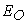= 25.30MN/mm2

Table A1 Calibration of model for normal concrete using equation 9

Substituting in equation 9, we have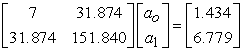Recalling the process for stress values of 0.50 and 1.00, the values of k and C are found as follows:From the above values of constants, C can be approximated to unity

Thus, for normal concrete equation 3 becomes: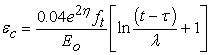(I)
B. Calibration of High Strength Concrete

For high strength concrete, the experimental results of tensile creep tests by K. Hans-Wolf Reinhardt, Tassilo Rinder  were used for calibrating the model. The optimization operations is presented in Table B1

Tensile strength= 5.20N/mm2

Elastic modulus= 45.50MN/mm2

Stress Level = 1.00

Table B1 Calibration of model for high strength concrete, using equation 9

Using equation 9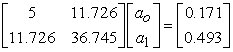From the above values of constants, k and C can be approximated to 0.01 and 1.00 respectively

Thus, for high strength concrete equation 3 becomes: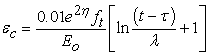(II)

APPENDIX 2COMPUTATION OF ERROR COEFFICIENT M FORNORMAL CONCRETEAPPENDIX 3 COMPUTATION OF ERROR COEFFICIENT M FOR HIGH STRENGTH CONCRETE